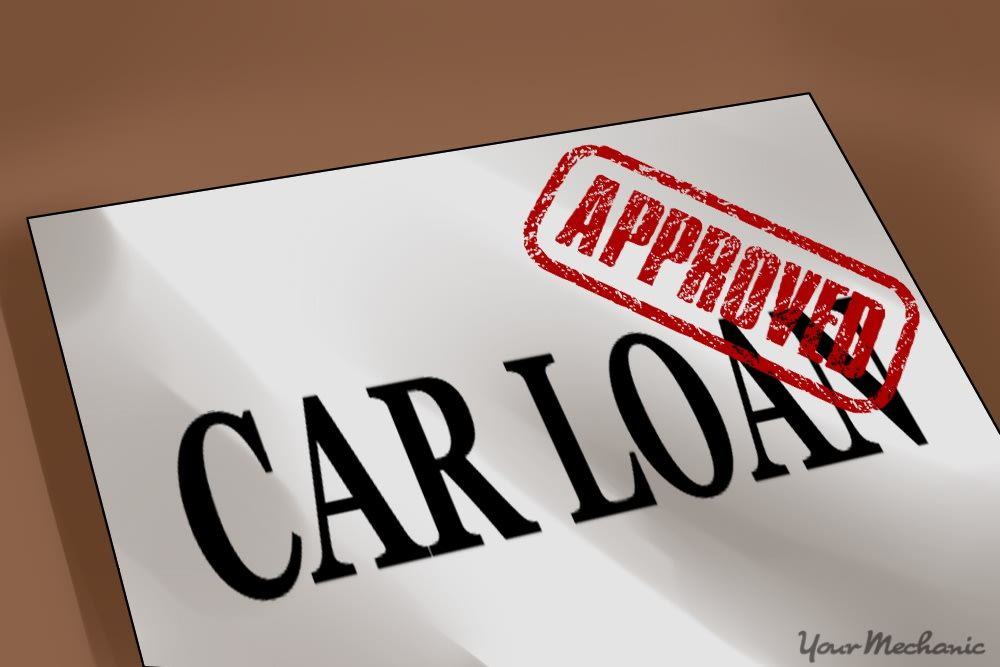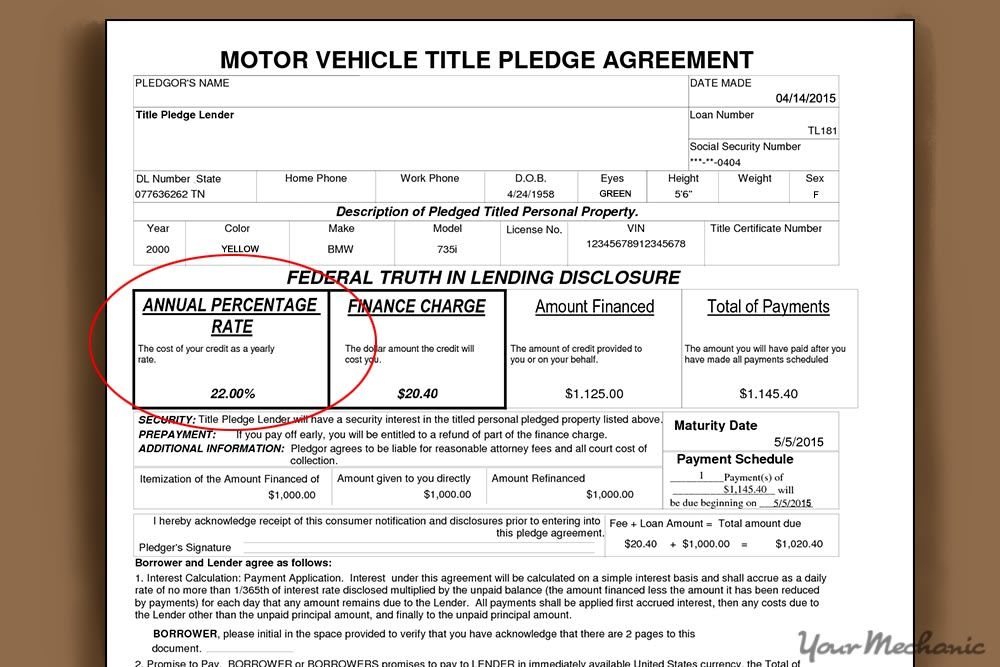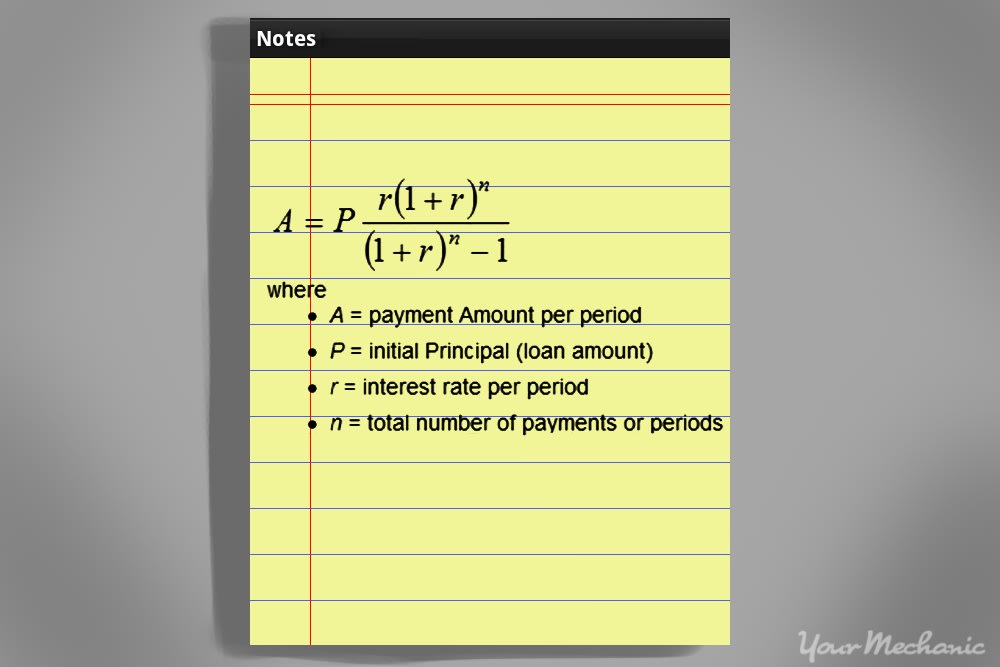# How to Determine the Total Interest Paid on a Car LoanSchedule Oil Change
Service Area

## \$92.73 - \$160.32

Price range for all vehicles
Average rating from 17,606 customers who received a Change Oil and Filter.An important aspect of any vehicle loan is the amount of interest you pay. If you want to compare loans to see which one is the better deal or just figure out how much interest you’ve paid in for the life of your car loan, you can calculate the total amount of interest paid. Follow these steps to know the true price of the vehicle you purchased.

## Part 1 of 4: Find out how much is being financed and for how long

The first step of the loan process is determining which loan you want to pursue. This requires you to figure out how much you can expect to pay in principal, annual percentage rate (APR), and other fees.

Step 1: Find out the principal. Discover the amount you are financing in your loan, which is called the principal.

It will be the purchase price minus any cash rebates (or other deductions) and your down payment and trade-in. When you subtract these amounts from the total purchase price, which will also include charges and the sales tax, it gives you the principal.

For example, if your car’s sticker price was \$20,000 and you traded in your old car for \$5,000 and gave \$2,000 as a down payment, your principal is \$13,000.

Step 2: Find out the length of the loan term. Many car loans for new or slightly used vehicles carry a term up to five or even six years. Some may go as long as seven years, but keep in mind that you pay more interest for longer-term loans.

## Part 2 of 4: Find the stated interest rate on your loan

After you determine the principal part of your loan, the next step in determining how much interest you will pay is to figure out the interest rate. The interest rate is only applied to the actual amount you borrow, so any other fees are not figured into that amount (except for taxes).Step 1: Find the APR section. Read your loan agreement where it will state the exact interest rate for your loan. It is listed as the APR or Annual Percentage Rate.

• Tip: Seek loans with a low APR. The lower the APR, the less you pay in interest over the lifetime of the loan. Most APRs range from 6 to 18%, depending on your credit score.

## Part 3 of 4: Calculate your total interest paid

Once you have the interest rate, it is time to calculate the total interest. Luckily, you can find free calculators online. Look for a vehicle interest calculator, as these take into account amortization, which means the principal and interest are reduced at different rates, with the interest being paid off more at the beginning of a loan and then gradually switching over to paying off more of the principal toward the end of the loan.Step 1: Use an online amortization calculator. This calculator takes the information you provide and figures how much interest is paid each month and for the life of the loan. You can find auto loan calculators online at such sites as Bankrate, Auto Loan Calculator, and Amortization Schedule Calculator.

Input your data, such as the principal loan amount, loan term, and interest rate. Some calculators allow you to look at the amortization schedule and add extra payments to see how they affect your overall payment schedule.

• Warning: Check with your lender before making any extra payments. Some put provisions in the loan paperwork stating that you will be penalized for paying off the loan early.

Step 2: Print or save the calculations you receive. Find the area that gives you the total amount of interest paid and highlight it.

• Tip: If you are considering financing or re-financing your car, you can input different scenarios to find out how to save on the interest you’ll pay. When re-financing, compare what different companies offer to find the best deal for you.

## Part 4 of 4: Calculate interest paid by hand

You can also calculate the total interest you can expect to pay by hand using a simple formula. Before doing so, make sure you have the information you need, including how much you pay per month, the principal loan amount, and the total number of payments you have to make.

Materials Needed

• Calculator
• Paper and pencilStep 1: Find the monthly interest. Divide the APR by 12 to determine the amount of monthly interest.

For our example we will assume that you have an APR of 6%. Divided by 12, this gives you a monthly interest rate of .5%. Convert this further into its decimal point equivalent, which is 0.005. As part of the equation, you have to add 1 to your monthly APR, giving you 1.005.

• Tip: You could opt to make a higher down payment to qualify for a lower APR. This reduces the total amount of interest you have to pay over the life of the loan.

Step 2: Factor in your other numbers. Further information you need for this equation includes the principal, or the amount you are borrowing, and the length of the loan, or the amount of time in months, that you plan on paying the loan back.

For this example, we will use \$15,000 as the amount of the principal, paid off over the course of five years, or 60 months.

Step 3: Raise the APR. For the top part of the equation raise the APR plus 1, or 1.005, to the power of 60, or the number of monthly payments you have to make. You do this by typing in the value in parentheses, 1.005, pressing the exponents button, denoted as x^y on your calculator, and then 60, which gives you 1.348.

Step 4: Multiply with the APR. Multiply the amount gained from the above step by the amount of the original APR, which in this case is 0.005. This gives you the sum of 0.00674.

Step 5: Add 1 to the APR. For the bottom part of the equation, add 1 to the APR per month of 0.005 to get 1.005. Enter 1.005 into your calculator, press the exponent button, x^y, and then press 60, or the number of payments, giving you a total of 1.348. Subtract 1 from this total to give you the sum of .348.

Step 6: Get the sum. Divide the numerator, 0.06674, by the denominator, .348 to get the sum of 0.0193. Multiply this by the amount of the principal, 15,000, giving you a monthly payment of \$289.50 per month.

Step 7: Calculate the total amount. Multiply the monthly payment amount of \$289.50 by the total number of payments you have to make, which is 60, giving you the amount \$17,370 that you have to pay back in total over the lifetime of the loan.

Step 8: Calculate the total interest. Subtract the principal of \$15,000 from the total amount you have to pay back, or \$17,370. This comes out to \$2,370, meaning you will pay that amount in interest over the lifetime of the loan.

Knowing how to figure the interest you can expect to pay over the lifetime of an auto loan helps you decide which loan is the right one for you. Seek out lenders who offer low APRs to get the best rates. Failing that, always work to keep your credit score high or pay a higher down payment to improve your APR and reduce the amount you have to pay back.

## Next Step

### Schedule Oil Change

Engine oil is the lifeblood of the engine. The oil resides in the oil pan, which is under the car attached to the bottom of the engine. All internal (moving) parts of the engine need to be lubricated by the engine oil. Inadequate lubrication will cause the parts to wear out faster and eventually lea... LEARN MORE

SEE PRICING & SCHEDULING

The statements expressed above are only for informational purposes and should be independently verified. Please see our terms of service for more details

### Need Help With Your Car?

Our certified mobile mechanics make house calls in over 2,000 U.S. cities. Fast, free online quotes for your car repair.

GET A QUOTE